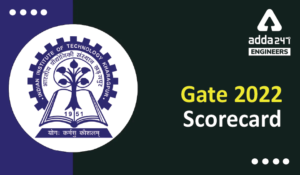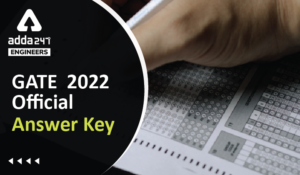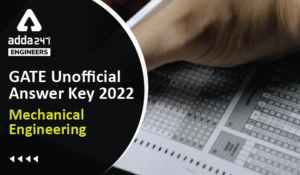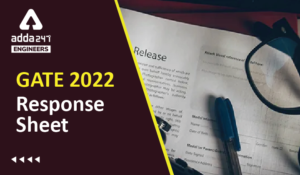Engineering Jobs   »   Civil Engineering Quiz

# GATE’22 CE:- Daily Practice Quiz 27-Aug-2021

IIT Kharagpur has released the GATE 2022 exam dates. As per the GATE 2022 exam date, the exam will be conducted on February 5, 6, 12 & 13. Candidates preparing for GATE 2022 must attempt this Civil Engineering Quiz to boost your GATE exam preparation.

Quiz: Civil Engineering
Exam: GATE
Topic: Miscellaneous

Each question carries 2 marks
Negative marking: 1/3 mark
Time: 12 Minutes

Q1. A 4-hour rainfall in a catchment of 250 sq.km, produces rainfall depth of 6.2 cm and 5.0 cm in successive 2-hour unit period. Assuming the ϕ – index of the soil to be 1.2 cm/hour, the runoff volume in hectare meter will be?
(a) 2200
(b) 1600
(c) 22
(d) 16

Q2. If the principal stresses at a point in a stressed body are 150 kN/m² tensile and 50 kN/m² compressive, then maximum shear stress at this point will be
(a) 250 kN/m²
(b) 150 kN/m²
(c) 100 kN/m²
(d) 50 kN/m²

Q3. The batching tolerance for cement as per IS 456 is
(a) ±1%
(b) ±1.5%
(c) ±2%
(d) ±3%

Q4. The optimistic, most likely, and pessimistic estimates of time for an activity are 4 days, 11 days and 12 days respectively. the expected completion time of this activity is
(a) 8 days
(b) 9 days
(c) 10 days
(d) 11 days

Q5. The concrete mix design is conducted as per
(a) IS: 383
(b) IS: 456
(c) IS: 10262
(d) IS: 13920

Solutions

S1. Ans.(b)
Sol.

 Time(hour) 0-2 2-4 Rainfall (Cm) 6.2 5.0

ϕ-index=1.2 cm\/hour
A=250 km^2=250×10^(6 ) m^2
We know,
ϕ-index=(P-Q)/t
Where,
P=Precipitation\Rainfall
t=Duration of rainfall
Q = Runoff
1.2=((6.2+5)-Q)/4
Q=11.2-4.8
▭(Q=6.4 cm)
Runoff volume = total runoff × Area of catchment
=6.4×10^(-2)×250×10^6
=1600×10^4 m^3
=1600 ha-m (∵1hecatare=10^(4 ) m^2 )

S2. Ans.(c)
Sol, Given,
σ_1= +150 kN\/m^2 (tensile)
σ_2= -50 kN\/m^2 (compressive)
τ=0
We know →
Maximum shear stress = Radius of Mohr circle
√(((σ_1-σ_2)/2)^2+τ^2 )
√([150-(-50)]^2/4+(0)^2 )
√((100)^2 )
=100 kN\/m^2

S3. Ans.(c)
Sol.
Material and their Batching tolerance limit –
Cement = ± 2%

S4. Ans.(c)
Sol. Given, Optimistic time (t_0 )=4
Most likely time (t_m )=11
Pessimistic time (t_P )=12
Expected time (t_E )= ?
We know,
t_E=(t_0+4 t_m+t_P)/6
=(4+(4×11)+12)/6
=60/6
▭(t_E=10 days)

S5. Ans.(c)
Sol. IS : 10262 → Concrete mix design
IS : 456 → Reinforced concrete
IS : 1343 → Prestress concrete

Sharing is caring!

Thank You, Your details have been submitted we will get back to you.
•HURL Recruitment 2022 Apply Online for 1...
•GATE 2022 Scorecard Released, Check Step...
•GATE Result 2022 OUT Live Check Your GAT...
•GATE 2022 Official Answer Key Out, Downl...
•GATE 2022 Answer Key Mechanical Engineer...
•GATE 2022 Response Sheet, Direct Link to...